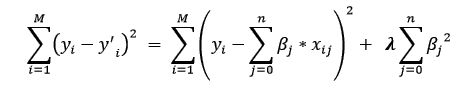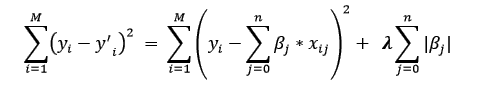# Regularization in Machine Learning

In this page we will learn about Regularization in Machine Learning, What is Regularization?, How does Regularization Work?, Techniques of Regularization, Ridge Regression, Lasso Regression, Key Difference between Ridge Regression and Lasso Regression.

## What is Regularization?

One of the most fundamental topics in machine learning is regularization. It's a method of preventing the model from overfitting by providing additional data.

The model performs well with the training data, but not with the test data. It means that the model is unable to anticipate the outcome when dealing with unknown data by injecting noise into the output, and as a result, the model is said to as overfitted. A regularization technique can be used to solve this problem.

This strategy can be applied in such a way that all variables or features in the model are preserved but the magnitude of the variables is reduced. As a result, the model's accuracy and generalization are preserved.

Its main function is to minimize or regularize the coefficient of features to zero. "In regularization technique, we reduce the magnitude of the features by keeping the same number of features." to put it simply.

## How does Regularization Work?

The complex model is regularized by introducing a penalty or complexity term. Consider the following equation for linear regression:

### y= β0 + β1x1 +β2x2 + β3x3 + ⋯ + βnxn + b

In the above equation, Y represents the value to be predicted

X1, X2, …Xn are the features for Y.

β0,β1,…..βn are the weights or magnitude attached to the features, respectively. The bias of the model is represented by a, and the intercept is represented by b.

To minimize the cost function, linear regression models strive to optimize the β0 and b. The cost function for the linear model has the following equation:We'll now add a loss function and an optimize parameter to create a model that can accurately forecast the value of Y. RSS, or Residual Sum of Squares, is the loss function for linear regression.

## Techniques of Regularization

There are mainly two types of regularization techniques, which are given below:

• Ridge Regression
• Lasso Regression

## Ridge Regression

• Ridge regression is one of the varieties of linear regression that introduces a little amount of bias to improve long-term predictions.
• Ridge regression is a model regularization technique that reduces the model's complexity. L2 regularization is another name for it.
• The cost function is changed in this method by including a penalty term. Ridge Regression penalty is the degree of bias introduced into the model. We may determine it by multiplying the squared weight of each individual feature by the lambda.
• In ridge regression, the cost function will be written as:• The penalty element in the above equation regularizes the model's coefficients, and so ridge regression reduces the amplitudes of the coefficients, reducing the model's complexity.
• As we can see from the equation above, if the values of λ tend to zero, the equation becomes the linear regression model's cost function. As a result, the model will approximate the linear regression model for the minimum value of λ.
• When there is a lot of collinearity between the independent variables, a general linear or polynomial regression will fail, then Ridge regression can be employed to tackle the problem.
• If we have more parameters than samples, it makes it easier to solve problems.

## Lasso Regression:

• Another regularization technique for reducing model complexity is lasso regression. Least Absolute and Selection Operator is what it stands for.
• It's similar to the Ridge Regression, but instead of a square of weights, the penalty term simply contains absolute weights.
• Because it uses absolute values, it can reduce the slope to 0, whereas Ridge Regression can only get close.
• L1 regularization is another name for it. The cost function of Lasso regression will be written as:• For model evaluation, some of the features in this technique are completely ignored.
• As a result, the Lasso regression can assist us in reducing model overfitting as well as feature selection.

## Key Difference between Ridge Regression and Lasso Regression

• Ridge regression is commonly used to reduce overfitting in models, and it takes into account all of the characteristics in the model. By decreasing the coefficients, it minimizes the model's complexity.
• Lasso regression aids in the reduction of model overfitting as well as feature selection.